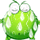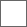• 77回复贴，共1

### data修改教程 by 剑笔小生其实都快忘记这个东西了，但是呢，看到很多同学还是对这个感兴趣，就把自己积累的一点东西发一发，主要是修改data.pkg和脚本文件的，高手就不要进来喷我了。

data部分的参数只能是有理数，这种无聊的东西是最简单的了随便讲讲……【accouterment.xml】
xml文件需要手动设定打开方式，最好是专业一点的编辑器，但是记事本足够使用，而且读取阅览比较快速方便，还请注意。
【范例全部使用五前的格式，小五的和五前差别之处会指出，但是一般没有】
<row 编号="4001" 名称="猫眼镜石" 图示id="3325" 角色="1023" 描述="能够将任何隐藏机关一眼识破的猫眼形宝石。" 作用="运势2，招架2。" 物攻="" 仙攻="" 防御="" 仙防="" 身法="" 运势="2" 命中="" 闪避="" 招架="2" field1="" 价格="300" 卖出丢弃="" field2="" 冻结抗性="" 中毒抗性="" 灼魔几率="" 混乱抗性="" 脱力抗性="" 麻痹抗性="" 沉默抗性="" 异变抗性="" 昏睡抗性="" 消极抗性="" 污浊抗性="" 即死抗性="" 水抗性="" 火抗性="" 土抗性="" 风抗性="" 雷抗性="" 阴抗性="" 阳抗性="" field3="" 水加成="" 火加成="" 土加成="" 风加成="" 雷加成="" 阴加成="" 阳加成="" field4="" 备注="" />

- - 别的都好解释了【clothes.xml】

【compose.xml】

<row 编号="1" 名称="紫菁玉蓉膏" 原料1="602" 数量1="1" 原料2="601" 数量2="2" 原料3="29" 数量3="4" 结果1="4" 结果2="17" />【flash.ini】fontinfo.ini

help.xml

journal.xml

【level.xml】【map.xml】

<row 地图id="21" 名称="司云崖" 脚本id="11002" 旗标id="16021" 地图1="1068" 地图2="1084" 地图3="" 地图4="" 地图5="" />【MapInfo.ini】【msteam】

<row 编号="1"

【music.lst】

3,map\003.mp3

[,]前面是赋予的代码，后面是游戏文件夹下→music（文件夹或者pkg文件）下面的路径，应该只支持标准的mp3和wav文件，这个有待测试

【mweapon.xml】

<row 编号="5001" 名称="酒葫芦" 模型id="401" 图示id="401" 角色="1" 描述="普通的葫芦，因为经常用来装酒所以带有一些酒香。" 作用="全属性提升2。" 物攻="2" 仙攻="2" 防御="2" 仙防="2" 身法="2" 运势="2" 命中="" 闪避="" 招架="" field1="" 价格="3000" 卖出丢弃="1" field2="" 冻结抗性="" 中毒抗性="" 灼魔几率="" 混乱抗性="" 脱力抗性="" 麻痹抗性="" 沉默抗性="" 异变抗性="" 昏睡抗性="" 消极抗性="" 污浊抗性="" field3="" 水抗性="" 火抗性="" 土抗性="" 风抗性="" 雷抗性="" 阴抗性="" 阳抗性="" field4="" 水加成="" 火加成="" 土加成="" 风加成="" 雷加成="" 阴加成="" 阳加成="" field5="" 备注="" />【npcdata】

<row 编号="1"
npc的编号，和怪物不冲突

x缩放="100" z缩放="100" y缩放="100" 特效缩放="100" 碰撞面积长="5" 碰撞面积宽="5"

【shoes】

【skill】

<row 编号="1" （技能的ID，脚本需要用）

mp="20" （气或者神的消耗量）【sound】

【state】

<row 编号="0"

【stringlist】

【stringList_sys】

【scriptlist.ini】

[item]
101=item001

data里面不少文件都有类似 脚本ID= 或者 ai脚本ID= 这样的字眼，那里的ID就可以到scriptlist.ini这里面寻找。也就是说，有了这个文件，寻找、修改或者新增都非常方便~skill.xml 里面的“斗志”你最后试出来没有啥意思
@大港口舰#### 扫二维码下载贴吧客户端